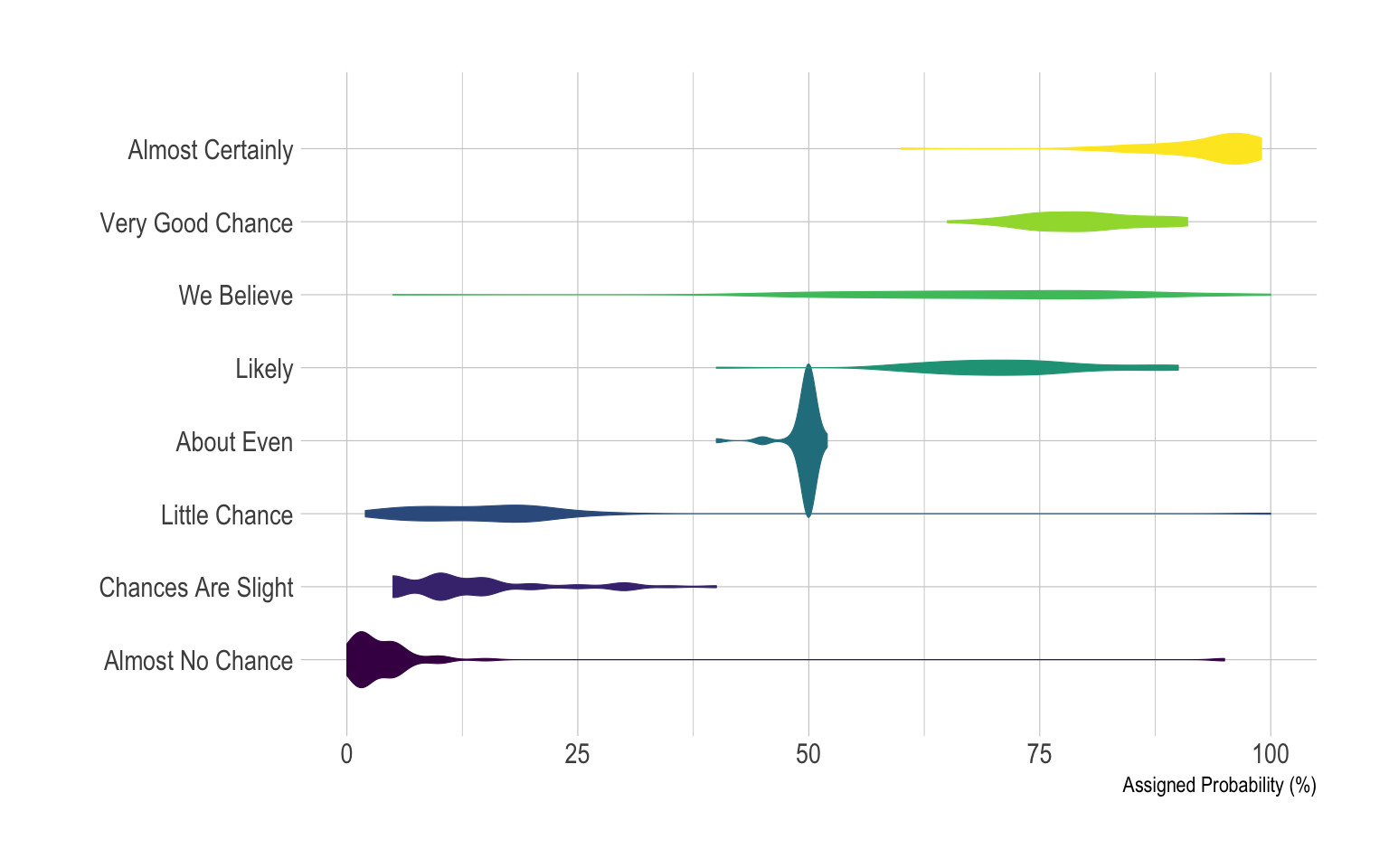# Horizontal violin plot with ggplot2

violin plots are useful to compare the distribution of several groups. Since group labels need to be read, it makes sense to build an horizontal version: labels become much more readable. This document provide an R implementation using ggplot2.

Building a violin plot with `ggplot2` is pretty straightforward thanks to the dedicated `geom_violin()` function. Here, calling `coord_flip()` allows to flip X and Y axis and thus get a horizontal version of the chart. Moreover, note the use of the `theme_ipsum` of the `hrbrthemes` library that improves general appearance.

``````# Libraries
library(ggplot2)
library(dplyr)
library(tidyr)
library(forcats)
library(hrbrthemes)
library(viridis)

# Data is at wide format, we need to make it 'tidy' or 'long'
data <- data %>%
gather(key="text", value="value") %>%
mutate(text = gsub("\\.", " ",text)) %>%
mutate(value = round(as.numeric(value),0)) %>%
filter(text %in% c("Almost Certainly","Very Good Chance","We Believe","Likely","About Even", "Little Chance", "Chances Are Slight", "Almost No Chance"))

# Plot
p <- data %>%
mutate(text = fct_reorder(text, value)) %>% # Reorder data
ggplot( aes(x=text, y=value, fill=text, color=text)) +
geom_violin(width=2.1, size=0.2) +
scale_fill_viridis(discrete=TRUE) +
scale_color_viridis(discrete=TRUE) +
theme_ipsum() +
theme(
legend.position="none"
) +
coord_flip() + # This switch X and Y axis and allows to get the horizontal version
xlab("") +
ylab("Assigned Probability (%)")

p``````Related chart types

## Contact

This document is a work by Yan Holtz. Any feedback is highly encouraged. You can fill an issue on Github, drop me a message on Twitter, or send an email pasting yan.holtz.data with gmail.com.